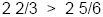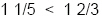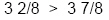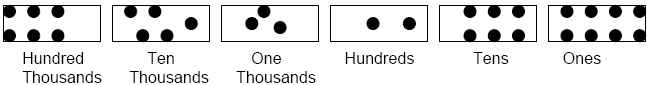Name:    2nd Grade Math Proficient Test 10

Multiple Choice
Identify the choice that best completes the statement or answers the question.

1.

Which comparison is true?
 a.d.b.e.c.2.

Number Sense and Numeration – Percents – RIT 191 – 200What percent is shown by this 100 unit figure?
 a. 9.5% d. 85% b. 95% e. 8.5% c. 850%

3.

1/4 = ____
 a. 20% d. 24% b. 25% e. 33% c. 30%

4.

Number Sense and Numeration – Place Value – RIT 191 – 200

What is 32 written as an ordinal?
 a. 3 tens, 2 ones d. thirty-two b. three-twos e. thirty-second c. thirtieth

5.

Put in expanded form: 406,219
 a. 400,000 + 60,000 + 200 + 10 + 9 d. 4,000 + 6,000 + 200 + 10 + 9 b. 46,000 + 200 + 10 + 9 e. 400,000 + 6,000 + 200 + 10 + 9 c. 40,000 + 6,000 + 200 + 10 + 9

6.

What number is named by four hundred thirty million, sixty-two thousand, four
hundred seven?
 a. 430,620,470 d. 462,407 b. 430,062,407 e. 430,602,407 c. 400,030,062,470

7.

The diagram below represents what number?a. 65,328 d. 563,268 b. 663,268 e. 635,628 c. 653,268

8.

Add and express in standard form.a. 117,630 d. 1,176 b. 1,763 e. 1,523 c. 11,763

9.

Number Sense and Numeration – Whole Numbers – RIT 191 – 200

Which number is greater than eight thousand nine hundred nine?
 a. 8,900 d. 8,800 b. 8.908 e. 80,000 c. 8,000

10.How many dozen doughnuts?
 a. 3 d. 2 1/2 b. 24 e. 4 c. 2

11.

Round 86,463 to the nearest ten thousand.
 a. 80,000 d. 90,000 b. 86,000 e. 86,400 c. 86,460

12.

Which are only prime numbers?
 a. 1, 3, 9, 12 d. 11, 17, 67, 19 b. 8, 16, 24, 36 e. 7, 49, 63, 77 c. 13, 23, 45, 68

13.

In which set are the numbers divisible by 2?
 a. 8, 10, 15 d. 20, 30, 45 b. 23, 37, 46 e. 9, 17, 23 c. 64, 90, 96

14.What is the missing factor in this factor tree?
 a. 6 d. 2 b. 8 e. 12 c. 9

15.

54 = 9 x 6
What is another way of naming 54?
 a. 2 x 3 x 9 d. 3 x 2 x 4 b. 3 x 3 x 2 x 2 e. 3 x 16 c. 26 x 2